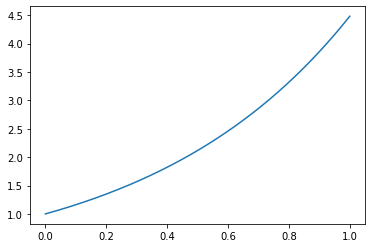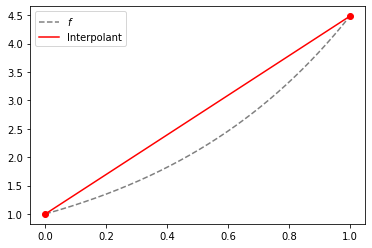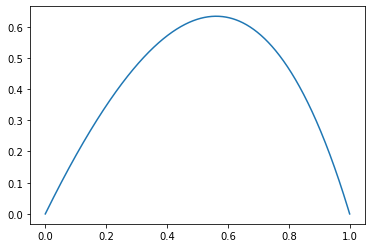# Interpolation Error¶

In :
import numpy as np
import numpy.linalg as la
import matplotlib.pyplot as pt


Let's fix a function to interpolate:

In :
if 1:
def f(x):
return np.exp(1.5*x)
elif 0:
def f(x):
return np.sin(20*x)
else:
def f(x):
return (x>=0.5).astype(np.int).astype(np.float)


In :
x_01 = np.linspace(0, 1, 1000)
pt.plot(x_01, f(x_01))

Out:
[<matplotlib.lines.Line2D at 0x11d896450>]And let's fix some parameters. Note that the interpolation interval is just $[0,h]$, not $[0,1]$!

In :
degree = 1
h = 1

nodes = 0.5 + np.linspace(-h/2, h/2, degree+1)
nodes

Out:
array([0., 1.])

Now build the Vandermonde matrix:

In :
V = np.array([
nodes**i
for i in range(degree+1)
]).T

In :
V

Out:
array([[1., 0.],
[1., 1.]])

Now find the interpolation coefficients as coeffs:

In :
coeffs = la.solve(V, f(nodes))


Here are some points. Evaluate the interpolant there:

In :
x_0h = 0.5+np.linspace(-h/2, h/2, 1000)

In :
interp_0h = 0*x_0h
for i in range(degree+1):
interp_0h += coeffs[i] * x_0h**i


Now plot the interpolant with the function:

In :
pt.plot(x_01, f(x_01), "--", color="gray", label="$f$")
pt.plot(x_0h, interp_0h, color="red", label="Interpolant")
pt.plot(nodes, f(nodes), "or")
pt.legend(loc="best")

Out:
<matplotlib.legend.Legend at 0x11d89e0d0>Also plot the error:

In :
error = interp_0h - f(x_0h)
pt.plot(x_0h, error)
print("Max error: %g" % np.max(np.abs(error)))

Max error: 0.633384• What does the error look like? (Approximately)
• How will the error react if we shrink the interval?
• What will happen if we increase the polynomial degree?